Electrical Engineering Paper Editing Services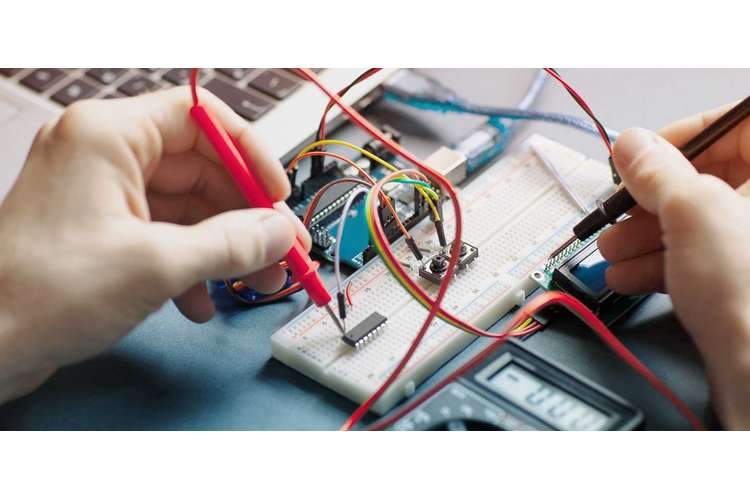Introduction

The control of the water level in the container was the main objective of the project. The project assisted in the analysis of the operation. The applied principles on these particular systems were exploited in other places apart from the water level. Some of the areas of the applications include the regulation of the temperatures and pressures. The level of water in the tank was monitored using a system of sensors. The sensor system has been configuring with the actuating system to make the operation of the management control system much easier. In the final stages of the project, the reaction curve method was used in the generation of the values of different variables for the PID controllers (Adamski et al 2013)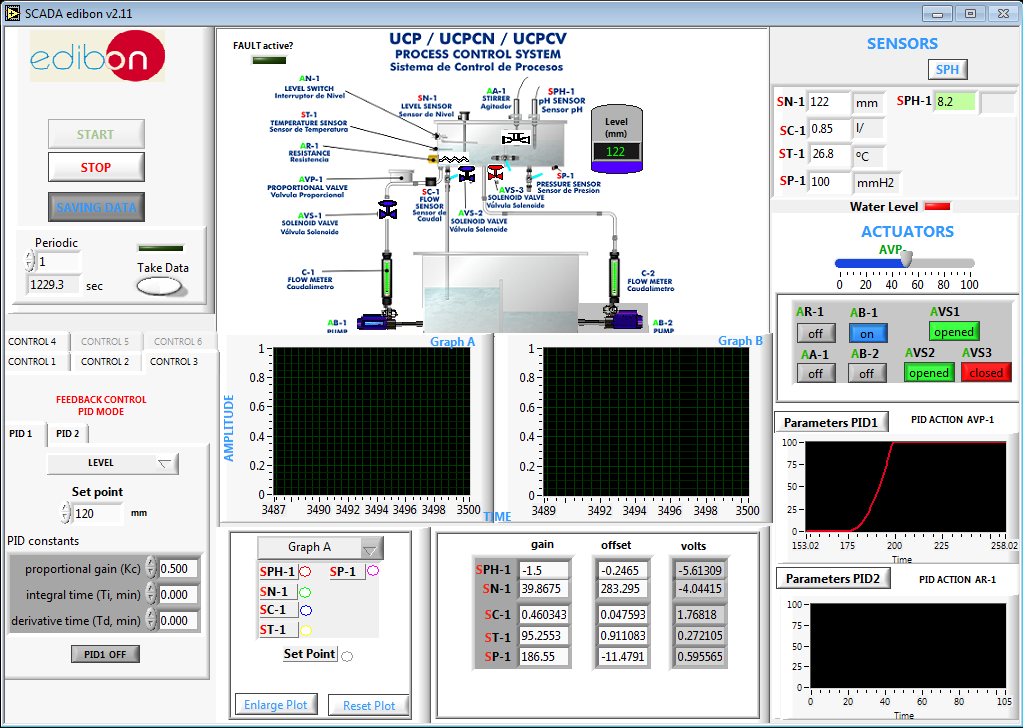Figure1. Edibon water level apparatus.

Aims

This laboratory project has an aim of understanding the basic principles in the controlling the liquid level in the tank by use of various control variables (Mathad et al 2013). The control output was adjusted to ensure that liquid bevel in the tank was brought the desired set point. This was done by the use of proportional (P), integral (I) and derivative (D) controllers in various combination. The main reason for using these principles was to control pressure, temperature and other variables as needed in different industries. The major reason for carrying out such experiments was to aid in the understanding of the crucial principles of controlling the liquid level within the tank while using variables of a different control. Through adjustment of the output of the control, the liquid level in the tank(Zammit, Staines and Apap 2014).

Level Control Loop (Proportional Control)

In a part of this experiment, the tank liquid level was controlled using a sensor and a configured controller for proportional output via the actuator. The configuration created an easier way of studying the system dynamics and applied control action responses. By means of the controller set which was proportional to output, the liquid level was regulated around 120mm set point. The actuator set at 20% initially. Though there should have been an increment of 10mm after the initial set reached and introduced to the initial set point band proportional controller (Kc) 0.5 parameter. The system feedback was given a careful observation while recording the result obtained. The experiment was repeated using two different values of Kc=o.25 and 0.75 for the achievement of different control responses. The system responses for three different Kc values that were obtained were as shown in figure 2 below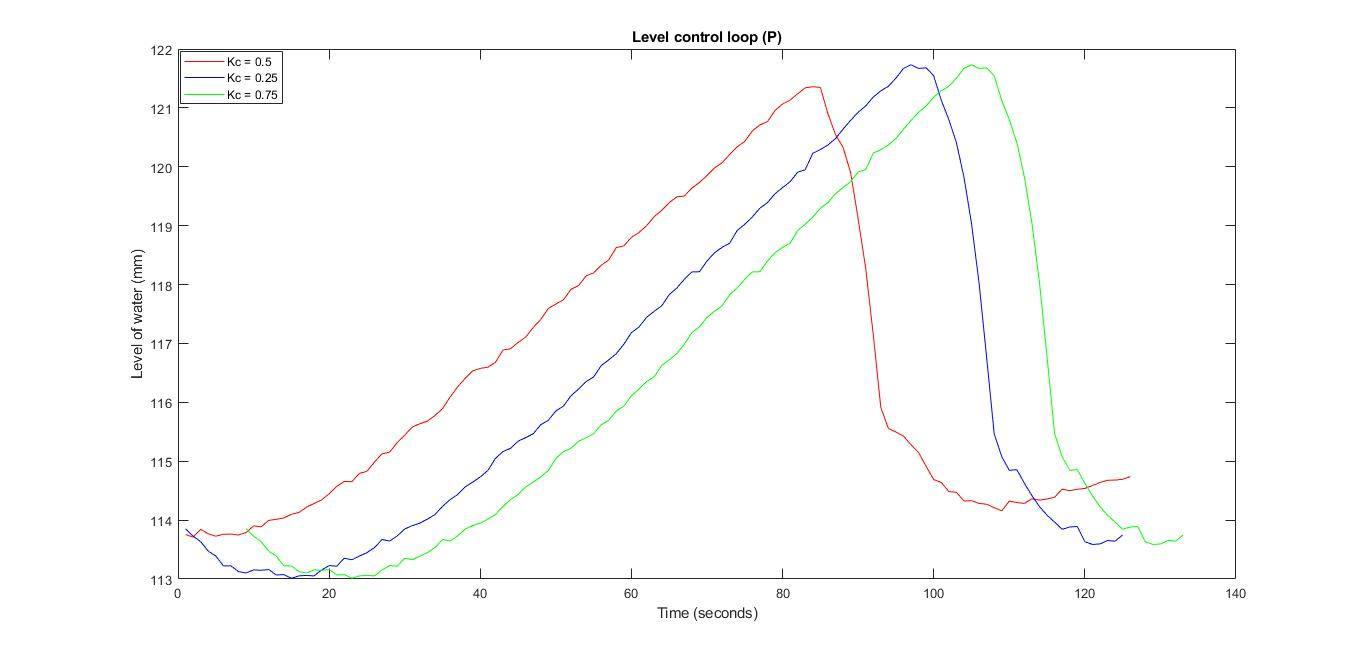Figure2. Level control using a proportional controller

The graph above shows the result obtained. The observation was made after tank water level was set at 114mm. There was 6mm water increment in the tank. This introduction was such that the new system was adjusted to a set point of (120mm) based on the proportional output (Kc=0.25, 0.5 and 0.75) through the system responses very slow. The level of water rises above the set point with a delay of approximately 60 sec at Kc=0.5 while with 80 sec at Kc= 0.25 and 0.75 before the system action to bring a level of water to the new set point was achieved. This was due to dead time creating laxity between the change in variable and time of the changes notification. In addition, the new setpoint variance was observed in the graph as an undershoot and overshoot.

Level Control Loop (PI Control)

In this particular part of the experiment, the liquid rise in the tank was monitored by use of sensor and a configured controller to ensure a proportional and integral actuator output. The value of proportional was set to 0.5 whereas that of integral varied between 0.1,5 and 10. The system response based on the various combination of proportional and integral control was observed and the result shown in figure 3. Besides this, in this laboratory, there was a clear observation of a lag time of 3 to 4 second between the actuator and the sensor in the apparatus.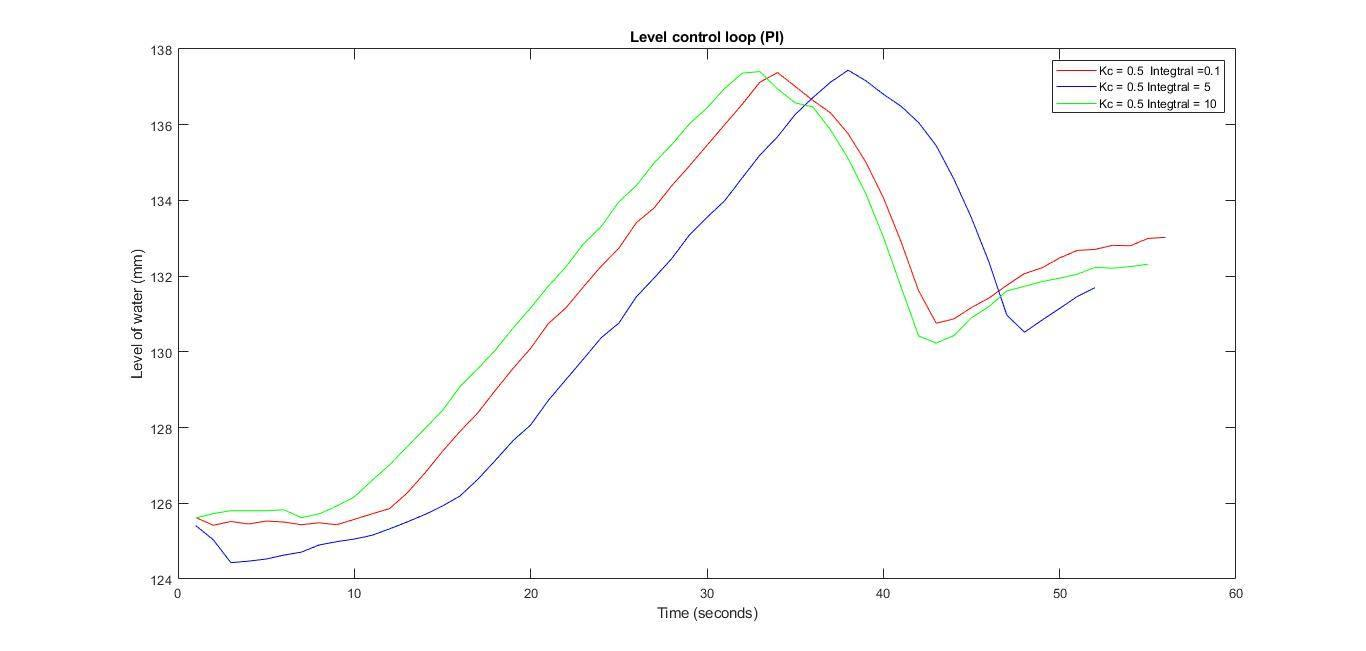Figure3. Level control using the PI controller

The observation from the graph above was that through the use of an integral controller additional with a proportional controller, the response of system has got better overall. The new setpoint variance (135ml) was less where the proportional and integral control set at 0.1 and 10 respectively. In other hand System also has got a quick response as compared to the previous laboratory. Conversely, if the proportional control value was set at 0.5 and the integral control was at 5, the slow response of the system observed with the setpoint variance was high. However, more time was taken for stabilization of the system as in the graph above (Mathad, Ronad and Jangamshetti 2013).

Level Control Loop (PD Control)

In this project section, the tank liquid rose under the regulation of sensor and configured the controller to produce proportional and derivative actuator output. The value of proportional was set to 0.5 and the values of derivative vary between 0.25, 0.5 and 0.75. The careful system response has done based on the various combination of controller output. In the laboratory, the derivative effect on the control system has more evidence with respect to the abrupt system adjustment to the new setpoint due to the introduction of the variance in the system. The obtained results were as shown in figure 4.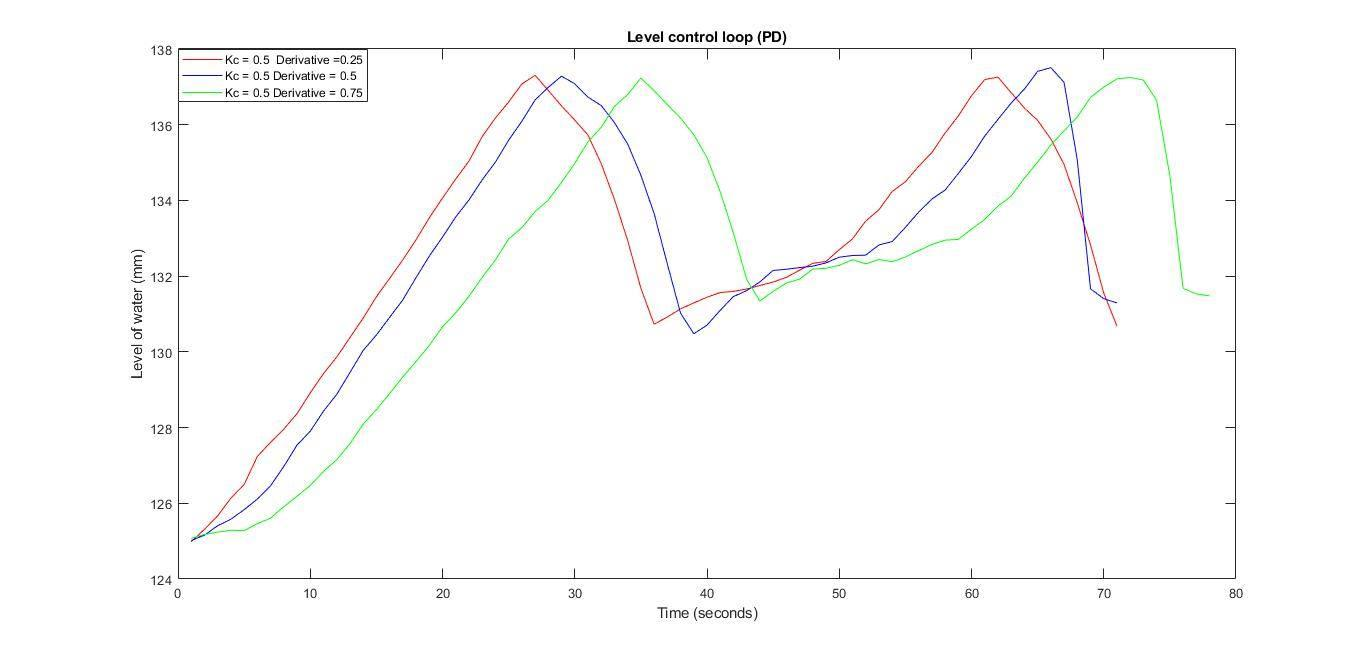Figure4. Level control using PD controller.

It is very clear from the above graph that the derivative controller value is increasing while the set variance from point (135mm) decreases significantly by –or + 3.3ml approximately. Besides, the system response was observed to be quicker the than two previous laboratories which were caused by the derivative control. The change recognized by a derivative takes a sudden action to ensure that the liquid level is brought to the desired setpoint (Mathad, Ronad and Jangamshetti 2013).

Level Control Loop (PID Control):

In this part of the experiment, the container liquid though controlled by a sensor and the controller configured to ensure proportional, derivative and integral actuator output. The proportional controller value varied between 0.25, 0.5 and 0.75 while those of integral controller varied between o.1, o.5 and 0.9 in this laboratory part, the integral and the derivative control was characterized by the combination and the proportional controller for the system output stabilization to ensure the desired level quickly while using less variance. The result obtained from this laboratory is shown in figure 5 below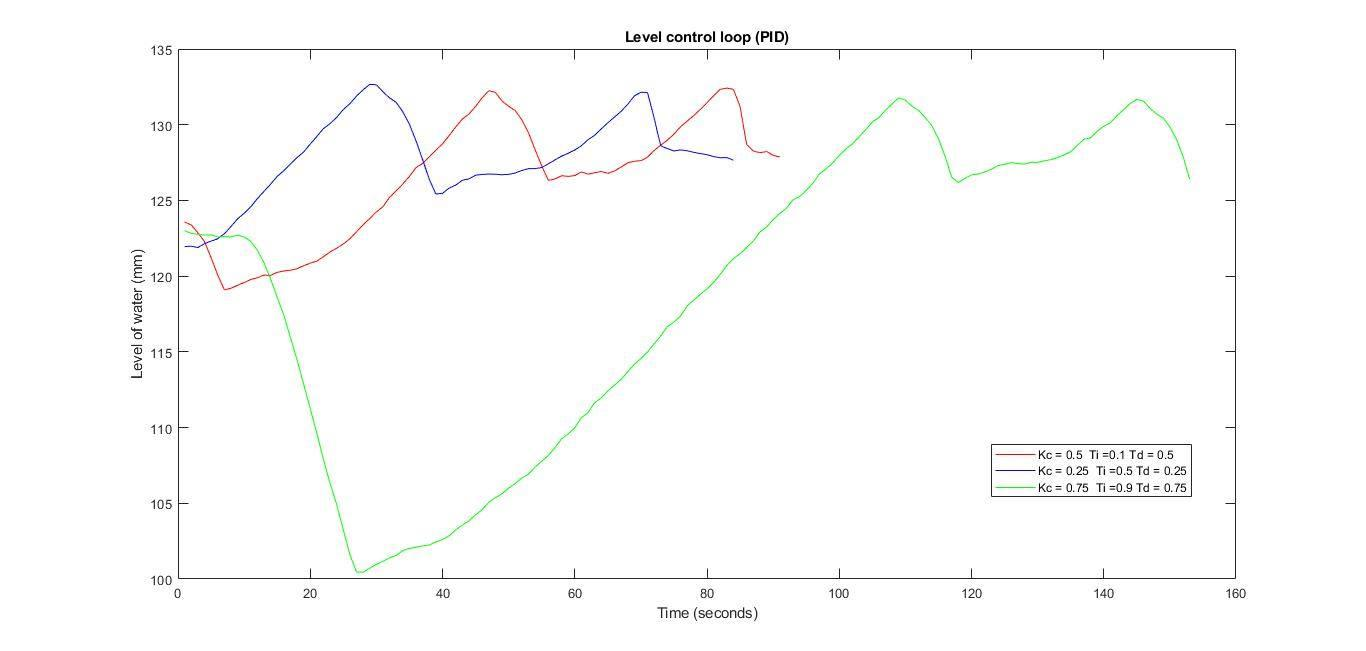Figure5. Level control using PID controller

The above graph is an evidence that the proportional controller value is 0.5, the value of integral is set at 0.1 while the function of the derivative is 0.5, and the system reaction is little slower as compared to the cases where proportional controller set at 0.25, Ti=0.5 and Td=0.25. in cases of a higher proportional controllervalue like 0.75, a higher integral value number Ti=0.9 and a higher derivative value Td=o.75 .is predicted in the system that variance slowly with according to adjustment( the water level 123 shuts the valve 20 to 0 until 100mm water level reached. At the same time, the opening of the valve continues to 100 till the level of water reached 2mm above the setpoint and shuts again at zero).

The observation was that the system response with PID controller was better compared to the other control combinations (Ortega, Perez, Nicklasson, and Sira 2013).

The response of the system increase with the introduction change and the water level variance decrease significantly

Reaction curve method

The reaction curve assisted in the determination of the of the maximum value.In this specific laboratory work, the controller that was used had the following features; integral controller part, the proportional and derivative components were used in a combination set up. The parameter of thesystem managementwas obtained through calculation using the relevant software. It is upon the opened loop that normally induces inputs into a quick exchange of the loop regulation. The results that were generated were very crucial in the induced system.

The process specifically involved tangent line tracing in the inflection of the sigmoid point and measure the value of R and L. The retard time (L) and the slope of tangent ras in the inflection of curve point were noted (Rus and Tolley 2015).

Retard time Tuning Control Loops (Reaction Curve method) referred to the time taken between the change instant in the step and the point of the tangent to the sigmoid cuts the former controlled variable value. With DP the percentage of the variable position in the control, valve aims in introduced the process steps as in the 6 below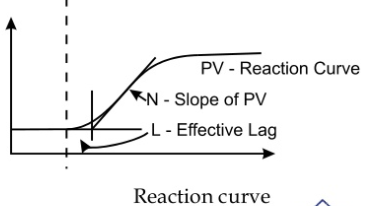Figure6. Reaction curve method.

The optimum controller PID values were got by the responses of the system in this section as shown in the figure 7 experiment. The introduction of a set of response and the tank water level allowed rising for a long time thus obtaining reaction curve, therefore facilitating the use of the reaction method allowing obtaining maximum PID controller figure. The tank water increment at a slow pace smoothed the reaction curve as obtained in figure 7 to be used further to determine the maximum of PID controller values.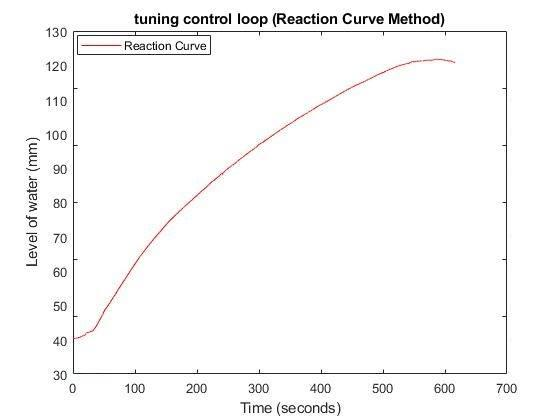Figure 7. Reaction curve from the system for tuning a control loop

The information in table 1 is used in the calculation of the highest PID controller figure

Values of xy of PID controller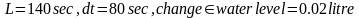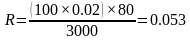•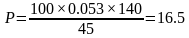•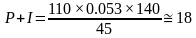With value based on the PID controller, the parameter obtained was set for integral, proportional and derivative control while carefully observing the response of the system. The obtained result of the values was recorded in figure 9 below.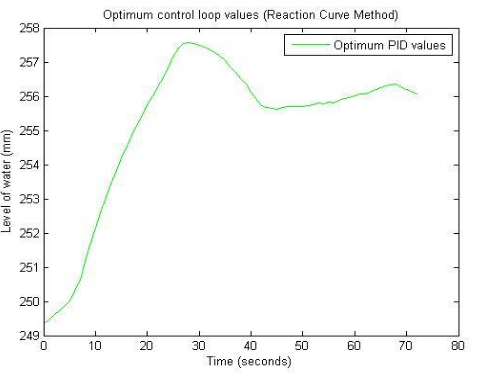Figure8. The response of the system with optimum PID values.

The PID controller optimum values in the above graph as obtained reaction method curve showed that the system recognizes a quick change while adjusting a new set point (120). The variance decreases from the set until the system reached the new set point that is observed in the graph above (after 40 seconds). The stability of the system remains intact condition after then a little variation that was only observed in the interval(Pimentel, Gonzalez and Barbosa 2016).

Conclusion

In order to conclude in the trial of the experiment, the response of a particular system of different configuring with varied output was analyzed.The level of water in the tank was allowed to rise to the point that had been already been set at 250mm. This was then followed by an increment of 5mm that was introduced at intervals. The observation was then made in order to establish the reaction of the system in response to the values of the control. The values that were obtained subsequently aided the production of the results. The results that beware obtained indicated that the response of the system was relatively much efficient when proportional, derivative and integral combinations were used in the process (Zammit et. al 2014)

At the end of the exercise, there was the use of the curve reaction to getting the maximum variables of the PID control. These variables were found very useful in the laboratories for the analysis of the response system. The observation made was that the response system was very quick when the PID control was put into use. It was also observed that the variance from the set point was very little. The psychology experiment was found to be very useful since the underlying principles could be applied in other sectors that include regulation of the temperatures and pressure.

References

1. Adamski, M., Wi?niewska, M., Wi?niewski, R., and Stefanowicz, ?., 2012. Application of hypergraphs to the reduction of the memory size in the microprogrammed controllers with address converter.Przegld Elektrotechniczny, (8), pp.134-136.C. Wind energy conversion systems-a technical review. J. Eng. Sci. Technol8(4), pp.493-507.
2. Mathad, V.G., Ronald, B.F. and Jangamshetti, S.H., 2013. Review on a comparison of FACTS controllers for power system stability enhancement.International Journal of Scientific and Research Publications3(3), pp.2250-315.
3. Ortega, R., Perez, J.A.L., Nicklasson, P.J. and Sira-Ramirez, H.J., 2013. Passivity-based control of Euler-Lagrange systems: mechanical, electrical and electromechanical applications. Springer Science & Business Media.
4. Rus, D. and Tolley, M.T., 2015. Design, fabrication, and control of soft robots.Nature521(7553), p.467.C. A new model for a PMSG-based wind turbine with yaw control.IEEE transactions on energy conversion28(4), pp.929-937.
5. Pimentel, B.S., Gonzalez, E.S. and Barbosa, G.N., 2016. Decision-support models for sustainable mining networks: Fundamentals and challenges. Journal of cleaner production112, pp.2145-2157.
6. Zammit, D., Staines, C.S. and Apap, M., 2014. Comparison between PI and PR current controllers in grid-connected PV inverters.WASET, International Journal of Electrical, Electronic Science and Engineering8(2).